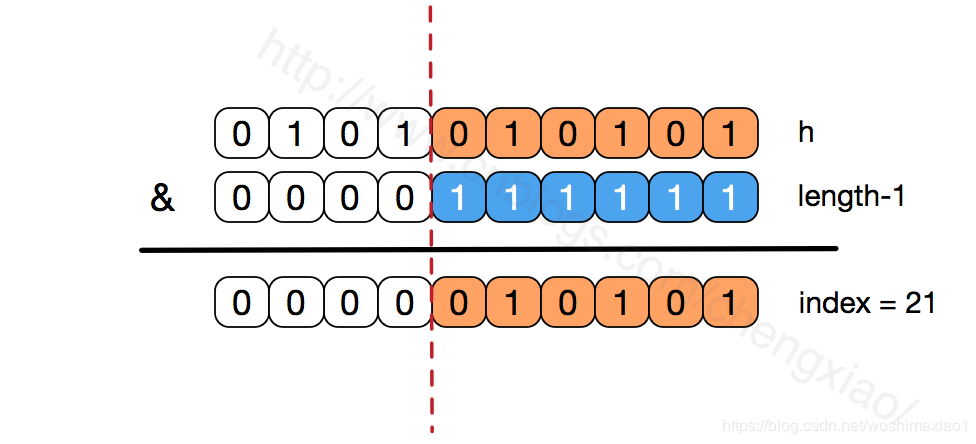﻿ JAVA中哈希表HashMap的深入学习_java_脚本之家
java# JAVA中哈希表HashMap的深入学习HashMap的主干是一个Entry数组。Entry是HashMap的基本组成单元，每一个Entry包含一个key-value键值对。（其实所谓Map其实就是保存了两个对象之间的映射关系的一种集合）

//HashMap的主干数组，可以看到就是一个Entry数组，初始值为空数组{}，主干数组的长度一定是2的次幂。
//至于为什么这么做，后面会有详细分析。
`transient Entry<K,V>[] table = (Entry<K,V>[]) EMPTY_TABLE;`
Entry是HashMap中的一个静态内部类。代码如下

``` static class Entry<K,V> implements Map.Entry<K,V> {
final K key;
V value;
Entry<K,V> next;//存储指向下一个Entry的引用，单链表结构
int hash;//对key的hashcode值进行hash运算后得到的值，存储在Entry，避免重复计算

/**
* Creates new entry.
*/
Entry(int h, K k, V v, Entry<K,V> n) {
value = v;
next = n;
key = k;
hash = h;
}
``````/**实际存储的key-value键值对的个数*/
transient int size;

/**阈值，当table == {}时，该值为初始容量（初始容量默认为16）；当table被填充了，也就是为table分配内存空间后，
int threshold;

/**负载因子，代表了table的填充度有多少，默认是0.75

*/

/**HashMap被改变的次数，由于HashMap非线程安全，在对HashMap进行迭代时，

transient int modCount;```

```public HashMap(int initialCapacity, float loadFactor) {
//此处对传入的初始容量进行校验，最大不能超过MAXIMUM_CAPACITY = 1<<30(230)
if (initialCapacity < 0)
throw new IllegalArgumentException("Illegal initial capacity: " +
initialCapacity);
if (initialCapacity > MAXIMUM_CAPACITY)
initialCapacity = MAXIMUM_CAPACITY;
throw new IllegalArgumentException("Illegal load factor: " +

threshold = initialCapacity;

}```

OK,接下来我们来看看put操作的实现

```public V put(K key, V value) {
//如果table数组为空数组{}，进行数组填充（为table分配实际内存空间），入参为threshold，
//此时threshold为initialCapacity 默认是1<<4(24=16)
if (table == EMPTY_TABLE) {
inflateTable(threshold);
}
//如果key为null，存储位置为table或table的冲突链上
if (key == null)
return putForNullKey(value);
int hash = hash(key);//对key的hashcode进一步计算，确保散列均匀
int i = indexFor(hash, table.length);//获取在table中的实际位置
for (Entry<K,V> e = table[i]; e != null; e = e.next) {
//如果该对应数据已存在，执行覆盖操作。用新value替换旧value，并返回旧value
Object k;
if (e.hash == hash && ((k = e.key) == key || key.equals(k))) {
V oldValue = e.value;
e.value = value;
e.recordAccess(this);
return oldValue;
}
}
modCount++;//保证并发访问时，若HashMap内部结构发生变化，快速响应失败
return null;
}```

inflateTable这个方法用于为主干数组table在内存中分配存储空间，通过roundUpToPowerOf2(toSize)可以确保capacity为大于或等于toSize的最接近toSize的二次幂，比如toSize=13,则capacity=16;to_size=16,capacity=16;to_size=17,capacity=32.

```private void inflateTable(int toSize) {
int capacity = roundUpToPowerOf2(toSize);//capacity一定是2的次幂
threshold = (int) Math.min(capacity * loadFactor, MAXIMUM_CAPACITY + 1);
table = new Entry[capacity];
initHashSeedAsNeeded(capacity);
}```

roundUpToPowerOf2中的这段处理使得数组长度一定为2的次幂，Integer.highestOneBit是用来获取最左边的bit（其他bit位为0）所代表的数值.

```private static int roundUpToPowerOf2(int number) {
// assert number >= 0 : "number must be non-negative";
return number >= MAXIMUM_CAPACITY
? MAXIMUM_CAPACITY
: (number > 1) ? Integer.highestOneBit((number - 1) << 1) : 1;
}```

hash函数

```/**这是一个神奇的函数，用了很多的异或，移位等运算

final int hash(Object k) {
int h = hashSeed;
if (0 != h && k instanceof String) {
return sun.misc.Hashing.stringHash32((String) k);
}

h ^= k.hashCode();

h ^= (h >>> 20) ^ (h >>> 12);
return h ^ (h >>> 7) ^ (h >>> 4);
}```

```/**
* 返回数组下标
*/
static int indexFor(int h, int length) {
return h & (length-1);
}```

h&（length-1）保证获取的index一定在数组范围内，举个例子，默认容量16，length-1=15，h=18,转换成二进制计算为index=2。位运算对计算机来说，性能更高一些（HashMap中有大量位运算）```void addEntry(int hash, K key, V value, int bucketIndex) {
if ((size >= threshold) && (null != table[bucketIndex])) {
resize(2 * table.length);//当size超过临界阈值threshold，并且即将发生哈希冲突时进行扩容
hash = (null != key) ? hash(key) : 0;
bucketIndex = indexFor(hash, table.length);
}

createEntry(hash, key, value, bucketIndex);
}```

```void resize(int newCapacity) {
Entry[] oldTable = table;
int oldCapacity = oldTable.length;
if (oldCapacity == MAXIMUM_CAPACITY) {
threshold = Integer.MAX_VALUE;
return;
}

Entry[] newTable = new Entry[newCapacity];
transfer(newTable, initHashSeedAsNeeded(newCapacity));
table = newTable;
threshold = (int)Math.min(newCapacity * loadFactor, MAXIMUM_CAPACITY + 1);
}```

```void transfer(Entry[] newTable, boolean rehash) {
int newCapacity = newTable.length;
//for循环中的代码，逐个遍历链表，重新计算索引位置，将老数组数据复制到新数组中去（数组不存储实际数据，所以仅仅是拷贝引用而已）
for (Entry<K,V> e : table) {
while(null != e) {
Entry<K,V> next = e.next;
if (rehash) {
e.hash = null == e.key ? 0 : hash(e.key);
}
int i = indexFor(e.hash, newCapacity);
//将当前entry的next链指向新的索引位置,newTable[i]有可能为空，有可能也是个entry链，如果是entry链，直接在链表头部插入。
e.next = newTable[i];
newTable[i] = e;
e = next;
}
}
}```

HashMap的数组长度一定保持2的次幂，比如16的二进制表示为 10000，那么length-1就是15，二进制为01111，同理扩容后的数组长度为32，二进制表示为100000，length-1为31，二进制表示为011111。从下图可以我们也能看到这样会保证低位全为1，而扩容后只有一位差异，也就是多出了最左位的1，这样在通过 h&(length-1)的时候，只要h对应的最左边的那一个差异位为0，就能保证得到的新的数组索引和老数组索引一致(大大减少了之前已经散列良好的老数组的数据位置重新调换)，个人理解。get方法：

``` public V get(Object key) {
//如果key为null,则直接去table处去检索即可。
if (key == null)
return getForNullKey();
Entry<K,V> entry = getEntry(key);
return null == entry ? null : entry.getValue();
}```

get方法通过key值返回对应value，如果key为null，直接去table处检索。我们再看一下getEntry这个方法

```final Entry<K,V> getEntry(Object key) {

if (size == 0) {
return null;
}
//通过key的hashcode值计算hash值
int hash = (key == null) ? 0 : hash(key);
//indexFor (hash&length-1) 获取最终数组索引，然后遍历链表，通过equals方法比对找出对应记录
for (Entry<K,V> e = table[indexFor(hash, table.length)];
e != null;
e = e.next) {
Object k;
if (e.hash == hash &&
((k = e.key) == key || (key != null && key.equals(k))))
return e;
}
return null;
} ```

```public class MyTest {
private static class Person{
int idCard;
String name;

public Person(int idCard, String name) {
this.idCard = idCard;
this.name = name;
}
@Override
public boolean equals(Object o) {
if (this == o) {
return true;
}
if (o == null || getClass() != o.getClass()){
return false;
}
Person person = (Person) o;
//两个对象是否等值，通过idCard来确定
return this.idCard == person.idCard;
}

}
public static void main(String []args){
HashMap<Person,String> map = new HashMap<Person, String>();
Person person = new Person(1234,"乔峰");
//put到hashmap中去
map.put(person,"天龙八部");
//get取出，从逻辑上讲应该能输出“天龙八部”
System.out.println("结果:"+map.get(new Person(1234,"萧峰")));
}
}

JDK1.8在JDK1.7的基础上针对增加了红黑树来进行优化。即当链表超过8时，链表就转换为红黑树，利用红黑树快速增删改查的特点提高HashMap的性能，其中会用到红黑树的插入、删除、查找等算法。